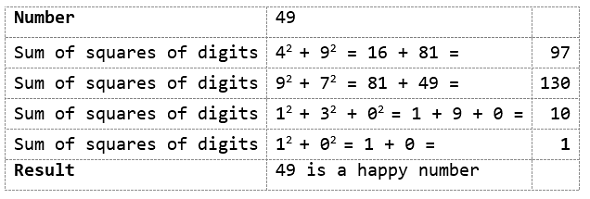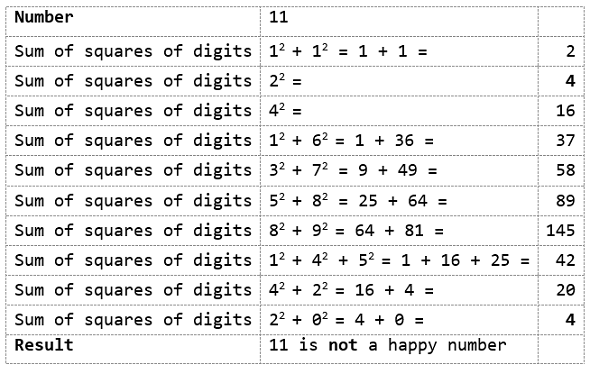# Python For GCSE Integer Sequences

## Introduction

This page should be read as a series of challenges. The challenge is to write a Python program that can produce the first n terms from the sequence that is described.

The sequences are listed in alphabetical order. There is no need to work through them in that order. Instead, write the code to produce them in whatever order you choose.

## Abundant Numbers

Abundant numbers are numbers for which the sum of the proper divisors of the number is greater than the number itself.

Proper divisors are the factors of a number, not including the number itself.

The first few abundant numbers are, 12, 18, 20, 24, 30, 36, 40, 42, 48, 54, 56, 60,...

## Automorphic Numbers

Automorphic numbers are numbers whose squares end with the same digit or digits as the number itself. To be clear, all of the digits of the starting number must be at the end of its square.

The first few automophic numbers are, 0, 1, 5, 6, 25, 76, 376, 625,...

## Deficient Numbers

A deficient number is a number for which the sum of the proper divisors is less than the number itself.

The first few deficient numbers are, 1, 2, 3, 4, 5, 7, 8, 9, 10, 11, 13, 14, 15, 16,...

## Fibonacci Numbers

Starting with 0 and 1, the sequence continues with each term being the sum of the previous 2 terms.

The sequence starts, 0, 1, 1, 2, 3, 5, 8, 13, 21, 34, 55, 89,...

## Happy Numbers

A number is happy if the process of summing the squares of the digits, taking the result and doing the same repeatedly, eventually ends with a sum of 1.In this second example, the sequence returns to 4. If the sum of the squares of the digits is ever one of these numbers, the number is not happy. For all unhappy numbers, this sequence will be reached somehow. Checking for a result of 4 is enough to determine that a number is not happy.

The early happy numbers are, 1, 6, 36, 44, 49, 79, 100, 160, 170, 216, 224,...

## Happy Prime Numbers

Happy prime numbers are happy numbers which are also prime. The first few are, 7, 13, 19, 23, 31, 79, 97, 103, 109, 139, 167, 193, 239, 263,...

## Harmonic Divisor Numbers

If you work out all of the factors of a number. Count how many factors there are. Divide that number by the sum of the reciprocals of those factors. If the result is an integer, the number is a harmonic divisor number.

The first ones are, 1, 6, 28, 140, 270, 496, 672, 1638,...

Harshad numbers are divisible by the sum of their digits. Like the following,

1, 2, 3, 4, 5, 6, 7, 8, 9, 10, 12, 18, 20, 21, 24, 27,...

## Highly Composite Numbers

Highly composite numbers have more factors than any other smaller integer. The first handful are,

1, 2, 4, 6, 12, 24, 36, 48, 60, 120, 180, 240, 360, 720, 840, 1260,...

If you plan on spitting out this sequence, make a list to store the number of factors as you go.

## Narcissistic Numbers

Narcissistic numbers are numbers which are equal to the sum of the digits of the number raised to a power equal to the number of digits. All single digit numbers are narcissistic.

The sequence begins with, 1, 2, 3, 4, 5, 6, 7, 8, 9, 153, 370, 371,... and there are only 89 such numbers in base 10. The largest one has 39 digits.

## Palindromic Numbers

Palindromic numbers are the same if their digits are reversed. Assume all single digit numbers to be palindromic.

0, 1, 2, 3, 4, 5, 6, 7, 8, 9, 11, 22, 33, 44, 55, 66, 77, 88, 99, 101,...

## Perfect Numbers

A perfect number is exactly equal to the sum of its proper divisors.

2, 3, 5, 7, 13, 17, 19, 31, 61, 89, 107, 127, 521, 607, 1279, 2203,...

## Permutable Prime Numbers

A permutable prime number is a number for which all permutations of its digits are prime numbers.

2, 3, 5, 7, 11, 13, 17, 31, 37, 71, 73, 79, 97, 113, 131, 199, 311, 337, ...

## Practical Numbers

A number is practical if its factors can be summed to produce all of the integers up to that number. You can use any number of the factors to make the sum. The trick to producing this sequence is being able to create a powerset from the list of factors. A powerset of a list is every possible sublist. For each integer up to the number, you can check if the sum of one of the sublists is equal to that integer.

1, 2, 4, 6, 8, 12, 16, 18, 20, 24, 28, 30, 32, 36, 40, 42, ...

## Prime Numbers

Prime numbers have exactly 2 factors, 1 and the number itself.

2, 3, 5, 7, 11, 13, 17, 19, 23, 29, 31, 37, 41, 43, 47, 53, 59,...

## Pronic Numbers

Pronic numbers are equal to the product of 2 consecutive integers and follow the sequence n(n+1). Pronic numbers can be arranged into rectangles of height n.

0, 2, 6, 12, 20, 30, 42, 56, 72, 90, 110, 132, 156,...

## Semiperfect Numbers

Semiperfect numbers are equal to the sum of all or some of its proper divisors.

6, 12, 18, 20, 24, 28, 30, 36, 40,...

## Triangular Numbers

Triangular numbers are so called because they count the objects that can form an equilateral triangle. The sequence starts with 0 or 1. To form the nth triangular number, you add n to the (n-1)th triangular number.

0, 1, 3, 6, 10, 15, 21, 28, 36, 45, 55, 66, ...

## Weird Numbers

Weird numbers are abundant numbers that are not semiperfect. The number is less than the sum of its proper divisors but no combination of those proper divisors can be summed to make the number.